Homework Help Question & Answers

# 3. Consider the following function: U=2x2-y2 (a) Find the stationary points (b) Find the saddle-point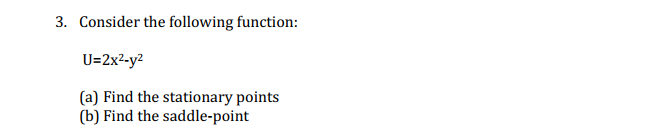3. Consider the following function: U=2x2-y2 (a) Find the stationary points (b) Find the saddle-point

#### Homework Answers

Answer #1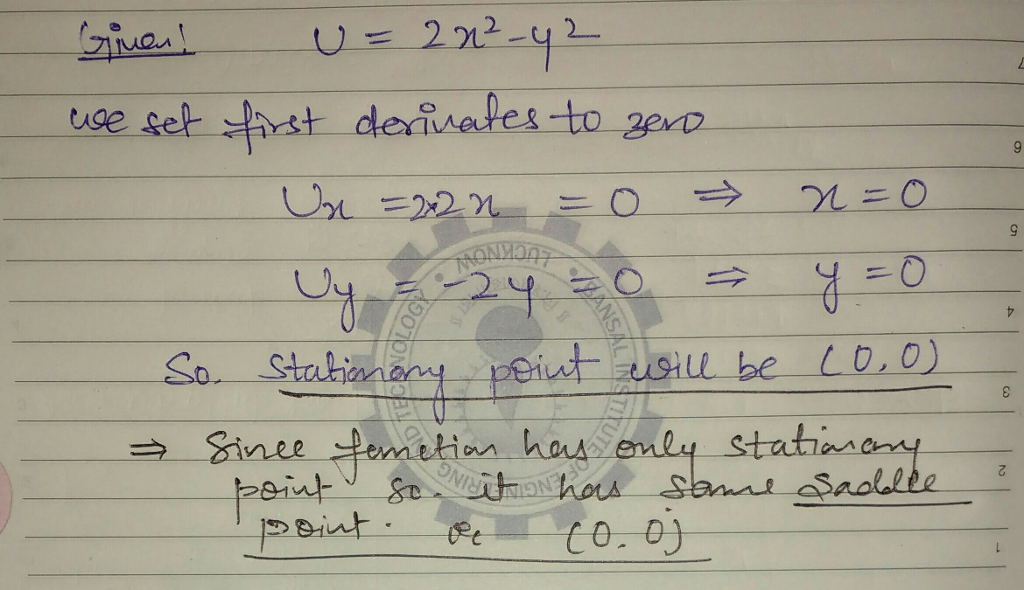Know the answer?
Your Answer:

#### Post as a guest

Your Name:

What's your source?

#### Earn Coin

Coins can be redeemed for fabulous gifts.

Not the answer you're looking for? Ask your own homework help question. Our experts will answer your question WITHIN MINUTES for Free.
Similar Homework Help Questions
• ### 5. [-13 Points) DETAILS TANAPCALC10 8.R.029. Consider the following. Ax,y) - 2x2 + y2 - 12x...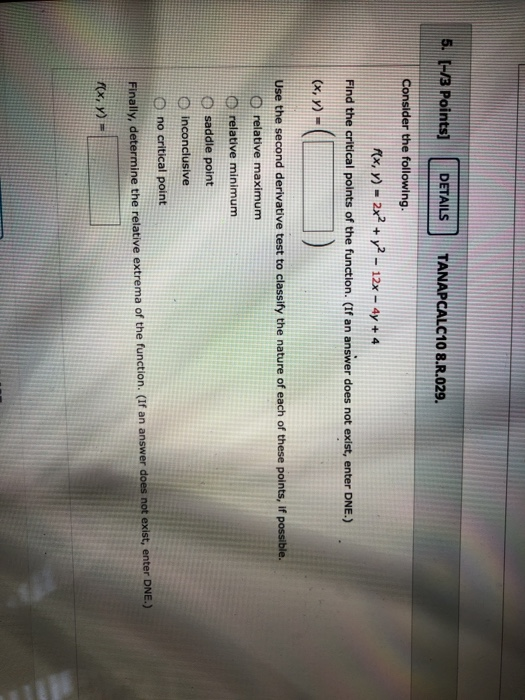5. [-13 Points) DETAILS TANAPCALC10 8.R.029. Consider the following. Ax,y) - 2x2 + y2 - 12x - 4y + 4 Find the critical points of the function. (If an answer does not exist, enter DNE.) (x, y) = Use the second derivative test to classify the nature of each of these points, if possible. O relative maximum relative minimum saddle point inconclusive no critical point Finally, determine the relative extrema of the function. (If an answer does not exist, enter...

• ### Find the local maximum and minimum values and saddle point(s) of the function. If you have...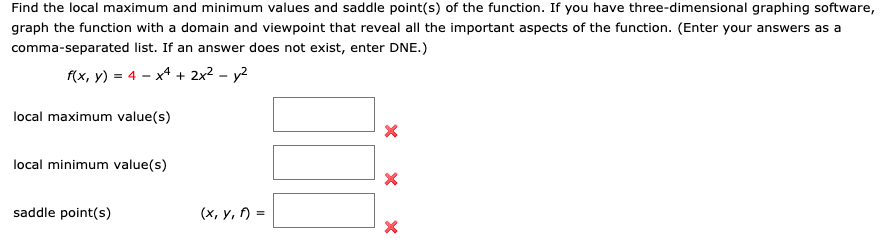Find the local maximum and minimum values and saddle point(s) of the function. If you have three-dimensional graphing software, graph the function with a domain and viewpoint that reveal all the important aspects of the function. (Enter your answers as a comma-separated list. If an answer does not exist, enter DNE.) f(x, y) = 4 − x4 + 2x2 − y2 local maximum value(s)      local minimum value(s)      saddle point(s)      (x, y, f) = Find the local maximum...

• ### |(a) Consider the following function for > 0 f (x)= = -4x 48x (i) Find the stationary point(s) of this function. (3...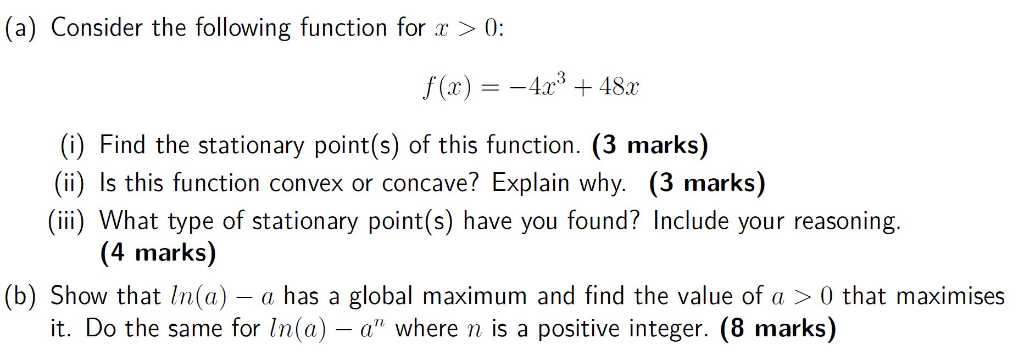|(a) Consider the following function for > 0 f (x)= = -4x 48x (i) Find the stationary point(s) of this function. (3 marks) (ii) Is this function convex or concave? Explain why. (3 marks) (iii What type of stationary point(s) have you found? Include your reasoning. (4 marks) |(b) Show that ln(a) - a has a global maximum and find the value of a > 0 that maximises it. Do the same for ln(a) - a" where n is a...

• ### Find all points where the function has any relative extrema or saddle points and identify the...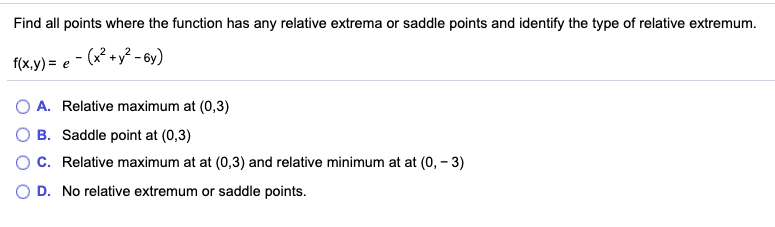Find all points where the function has any relative extrema or saddle points and identify the type of relative extremum. f(x,y)= e-(x2 + y2 -by) A. Relative maximum at (0,3) OB. Saddle point at (0,3) O C. Relative maximum at at (0,3) and relative minimum at at (0, -3) OD. No relative extremum or saddle points.

• ### Find the local maximum and minimum values and saddle point(s) of the function. If you have...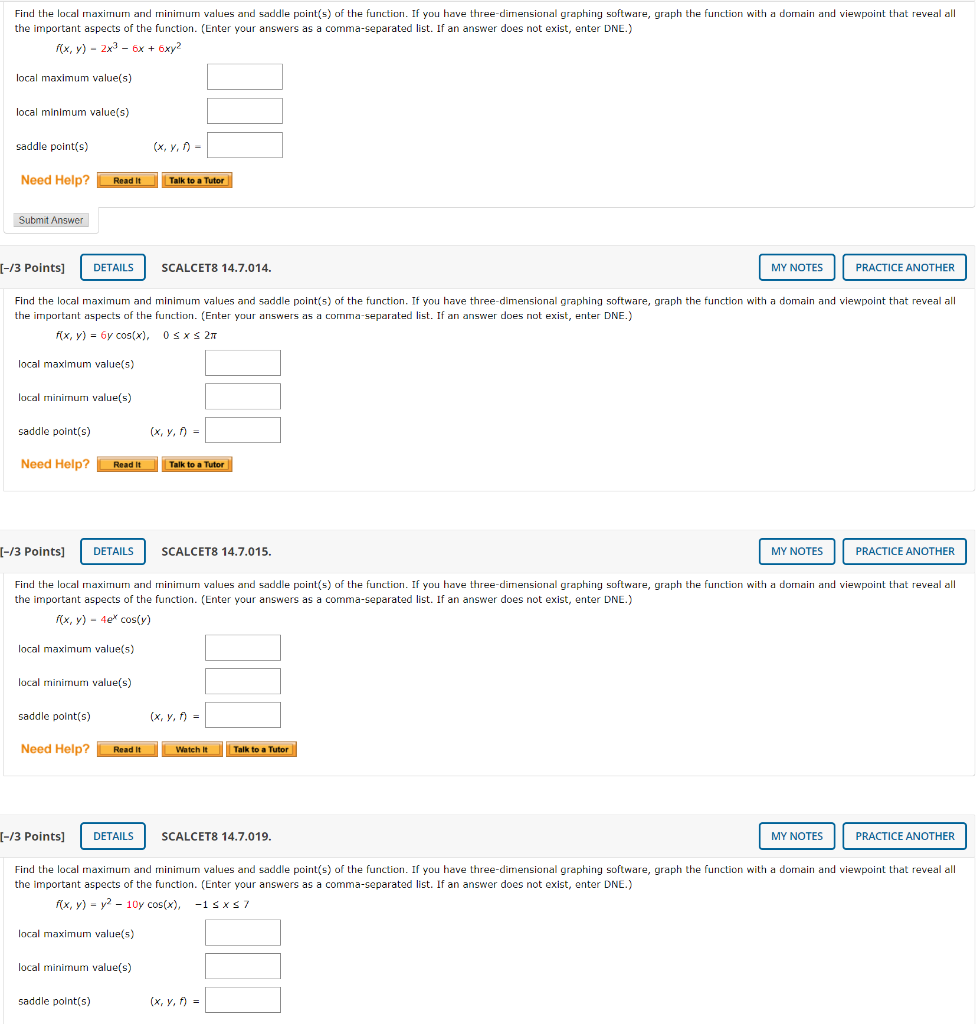Find the local maximum and minimum values and saddle point(s) of the function. If you have three-dimensional graphing software, graph the function with a domain and viewpoint that reveal all the important aspects of the function. (Enter your answers as a comma-separated list. If an answer does not exist, enter DNE.) f(x,y) - 2x2 - 6x + 6xy2 local maximum value(s) local minimum value(s) saddle points) Need Help? Read it Talk to a Tutor Submit Answer (-/3 points) DETAILS SCALCET8...

• ### 19. Find the critical points, relative extrema, and saddle points of the function. a. f(x, y)...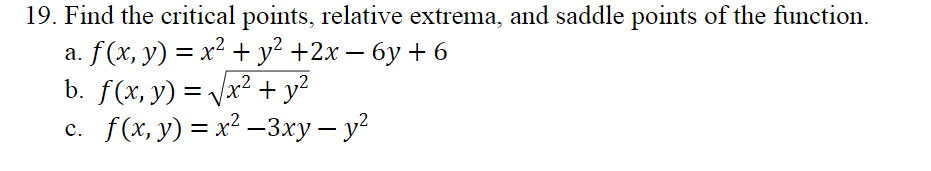19. Find the critical points, relative extrema, and saddle points of the function. a. f(x, y) = x2 + y2 +2x – 6y + 6 b. f(x, y) + y2 c. f(x, y) = x2 – 3xy - y2 = x²

• ### 3. Consider the following LP. Maximize u = 4x1 + 2x2 subject to X1 + 2x2...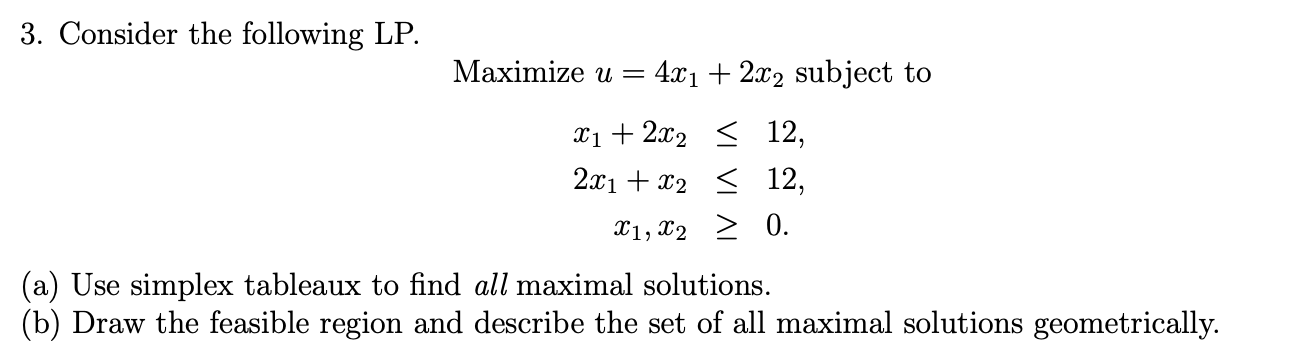3. Consider the following LP. Maximize u = 4x1 + 2x2 subject to X1 + 2x2 < 12, 2x1 + x2 = 12, X1, X2 > 0. (a) Use simplex tableaux to find all maximal solutions. (b) Draw the feasible region and describe the set of all maximal solutions geometrically.

• ### (17) Consider the function f that is given by f(x, y)-2y +e Find all its critical points and classify each one as a local maximum, local minimum, or saddle point (17) Consider the function f tha...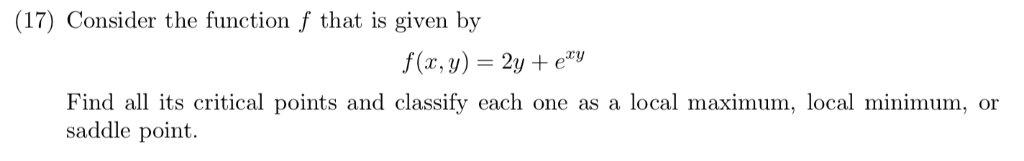(17) Consider the function f that is given by f(x, y)-2y +e Find all its critical points and classify each one as a local maximum, local minimum, or saddle point (17) Consider the function f that is given by f(x, y)-2y +e Find all its critical points and classify each one as a local maximum, local minimum, or saddle point

• ### 3. Show that the function f(x, y) = V26 – 2x2 - y2 is continuous at...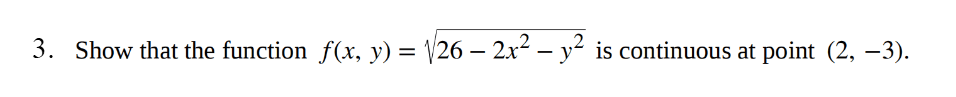3. Show that the function f(x, y) = V26 – 2x2 - y2 is continuous at point (2, -3).

• ### Problem #10: Consider the following function. 8(x,y) = {2x2 – 3y2 +6V6 y (a) Find the...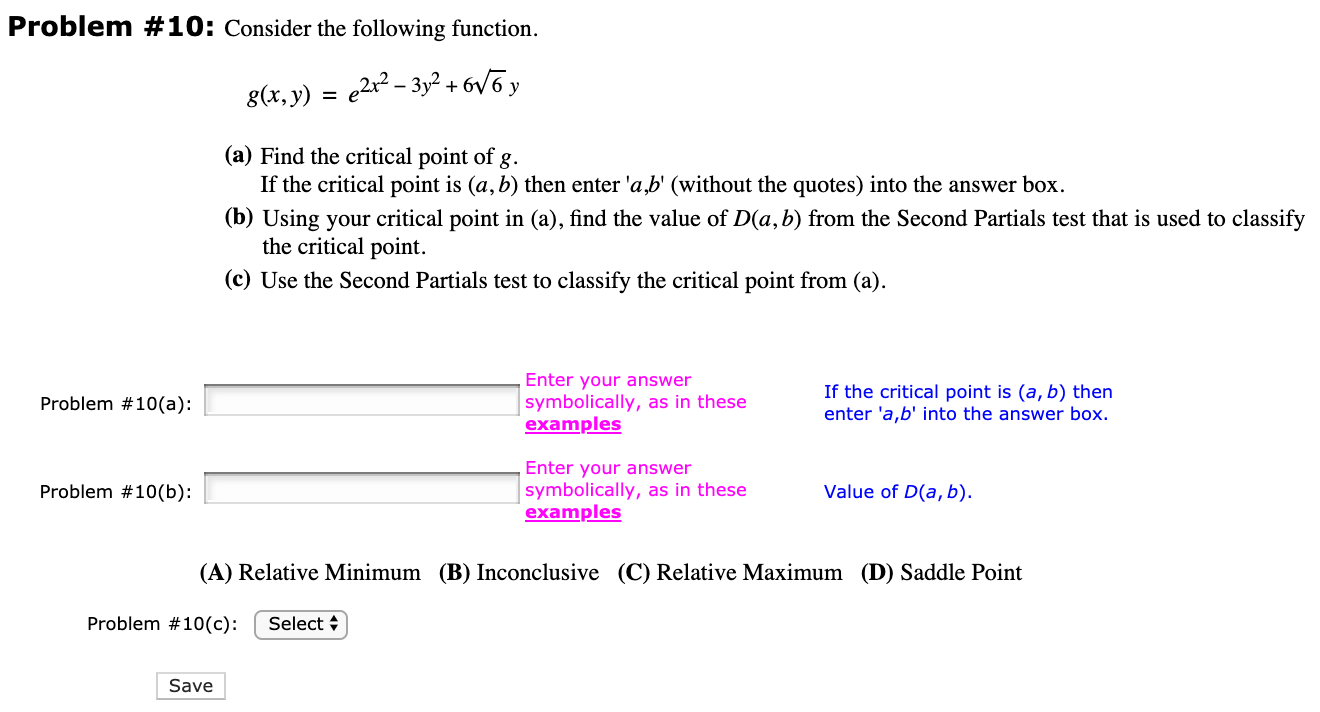Problem #10: Consider the following function. 8(x,y) = {2x2 – 3y2 +6V6 y (a) Find the critical point of g. If the critical point is (a, b) then enter 'ab' (without the quotes) into the answer box. (b) Using your critical point in (a), find the value of D(a,b) from the Second Partials test that is used to classify the critical point. (c) Use the Second Partials test to classify the critical point from (a). Problem #10(a): Enter your answer...

Free Homework App

Scan Your Homework
to Get Instant Free Answers
Need Online Homework Help?

Get Answers For Free
Most questions answered within 3 hours.